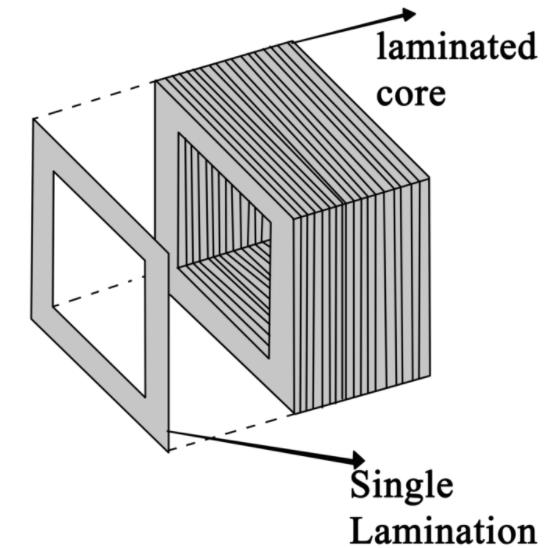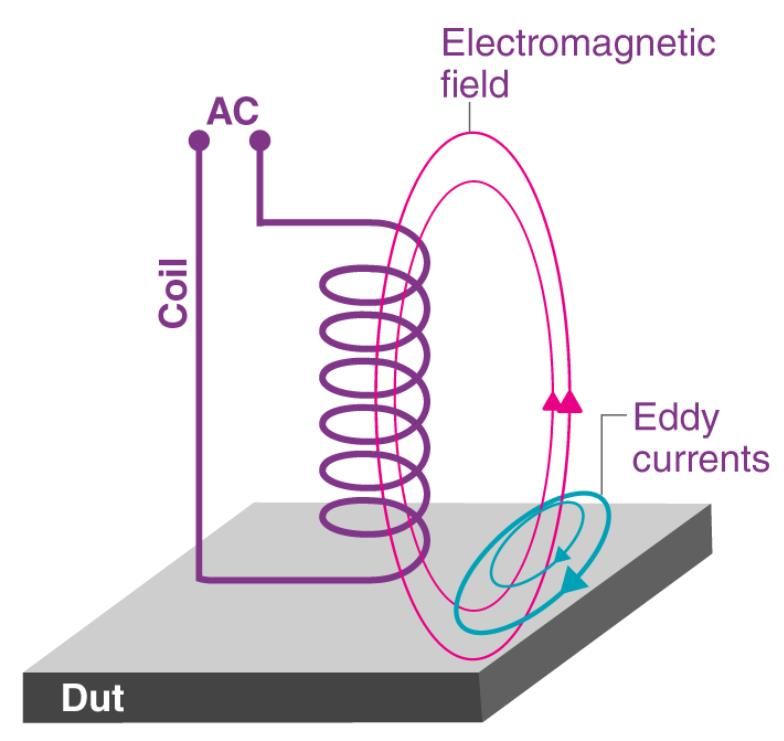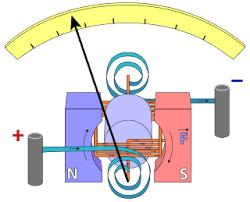Courses

# Test: Eddy Currents

## 15 Questions MCQ Test Physics For JEE | Test: Eddy Currents

Description
This mock test of Test: Eddy Currents for JEE helps you for every JEE entrance exam. This contains 15 Multiple Choice Questions for JEE Test: Eddy Currents (mcq) to study with solutions a complete question bank. The solved questions answers in this Test: Eddy Currents quiz give you a good mix of easy questions and tough questions. JEE students definitely take this Test: Eddy Currents exercise for a better result in the exam. You can find other Test: Eddy Currents extra questions, long questions & short questions for JEE on EduRev as well by searching above.
QUESTION: 1

### Why is the iron core of a transformer made laminated instead of being in one solid piece?

Solution:
• The iron core of a transformer is made of Laminated silicon steel with special insulated coating to limit Eddy current loss.
• Electrical steel, also called lamination steel is specialty steel tailored to produce certain magnetic properties, such as a small hysteresis area (small energy dissipation per cycle, or low core loss) and high permeability.QUESTION: 2

### Eddy currents do not cause:

Solution:

Eddy current when produced it causes damping heat loss energy loss but it don't produce any type of sparking.

QUESTION: 3

### Foucault Currents are also called

Solution:

Eddy currents are the currents which are induced in a conductor whenever the amount of linked magnetic flux with the conductor changes. These were discovered by Foucault in the year 1895 and hence they are also called Foucault currents.
Eddy currents:QUESTION: 4

Eddy currents have negative effects. Because they produce

Solution:
• When a conductive material is subjected to a time-varying magnetic flux, eddy currents are generated in the conductor.
• These eddy currents circulate inside the conductor generating a magnetic field of opposite polarity as the applied magnetic field. The interaction of the two magnetic fields causes a force that resists the change in magnetic flux.
• However, due to the internal resistance of the conductive material, the eddy currents will be dissipated into heat and the force will die out. As the eddy currents are dissipated, energy is removed from the system, thus producing a damping effect.
QUESTION: 5

The no-load current drawn by transformer is usually what percent of the full-load current ?

Solution:

The no load current is about 2-5% of the full load current and it accounts for the losses in a transformer. These no-load losses include core(iron/fixed) losses, which contains eddy current losses & hysteresis losses and the copper(I2R) losses due to the no Load current.

QUESTION: 6

Which of the following will not increase the size and effect of eddy current?

Solution:

Stronger magnetic field, thicker material and low resistivity material will increase the size and effect of eddy current whereas thinner material will reduce the effect of eddy currents.

QUESTION: 7

A circular coil of area 200 cm2 and 25 turns rotates about its vertical diameter with an angular speed of 20 ms-1 in a uniform horizontal magnetic field of magnitude 0.05 T. The maximum voltage induced in the coil is​:

Solution:

It is induced EMF of periodic EMI, So formula is:
E= NBAw
Here w is angular speed.

So, E = 25×0.05×200×20/10000 =0.5V

QUESTION: 8

Which of the following is not an application of eddy currents?

Solution:

Although eddy currents are undesirable, still they find applications in the following devices: Induction furnace, Electromagnetic damping, Electric brakes, Speedometers, Induction motor, Electromagnetic shielding, and energy meters.

QUESTION: 9

In electromagnetic induction, line integral of induced field E around a close path is __________, induced electric field is ___________.​

Solution:

In electromagnetic induction, line integral of induced field E around a close path is non zero, and induced electric field is non conservative.

QUESTION: 10

Identify the law which is used to find out the direction of eddy currents.

Solution:

Eddy currents are the currents induced in solid masses when the magnetic flux threading through them changes. Eddy currents also oppose the change in magnetic flux, so their direction is given by Lenz’s law.

QUESTION: 11

In the dead beat galvanometer, the coil is wound on a frame made of:​

Solution:

Aluminum is used as a coil which is a good conductor.QUESTION: 12

Identify the type of commercial motor which works as a consequence of eddy currents.

Solution:

A rotating magnetic field is produced employing two single-phase currents. A metallic rotor placed inside the rotating magnetic field starts rotating due to large eddy currents produced in it. These motors are commonly used in fans.

QUESTION: 13

Eddy currents can be induced in​

Solution:

Eddy currents are produced by varying magnetic field in solid plates, blocks and wires.

QUESTION: 14

Name the current induced in solid metallic masses when the magnetic flux threading through them changes.

Solution:

Eddy currents are the currents induced in solid metallic masses when the magnetic flux threading through them changes. These currents look like eddies or whirlpools in water and so they are known as eddy currents.

QUESTION: 15

How can electromagnetic damping be increased?

Solution:

The electromagnetic damping can be increased by winding the coil on a lighter copper or aluminum frame. As the frame moves in the magnetic field, eddy currents are set up in the frame which resists the motion of the coil.

• Test